Next: Exercises Up: Simple Harmonic Oscillation Previous: Simple Pendula

# Compound Pendula

Consider an extended body of mass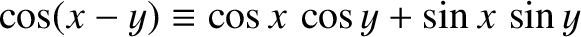with a hole drilled though it. Suppose that the body is suspended from a fixed peg, which passes through the hole, such that it is free to swing from side to side, as shown in Figure 6. This setup is known as a compound pendulum.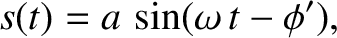Let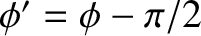be the pivot point, and letbe the body's centre of mass, which is located a distance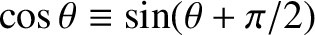from the pivot. Let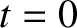be the angle subtended between the downward vertical (which passes through point) and the line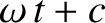(which is assumed to be confined to a vertical plane). The equilibrium state of the compound pendulum corresponds to the case in which the centre of mass lies vertically below the pivot point: that is,. The angular equation of motion of the pendulum is(55)

whereis the moment of inertia about the pivot, and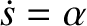is the torque. Using similar arguments to those employed for the case of the simple pendulum (recalling that the weight of the pendulum acts at its centre of mass), we can write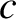(56)

The reaction,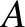, at the peg does not contribute to the torque, because its line of action passes through the pivot. Combining the previous two equations, we obtain the following angular equation of motion of the pendulum,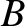(57)

Finally, adopting the small angle approximation,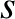, we arrive at the simple harmonic oscillator equation,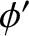(58)

By analogy with our previous solutions of such equations, the angular frequency of small amplitude oscillations of a compound pendulum is given by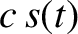(59)

It is helpful to define the length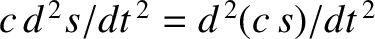(60)

Equation (59) reduces to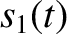(61)

which is identical in form to the corresponding expression for a simple pendulum. We conclude that a compound pendulum behaves like a simple pendulum with the effective length.Next: Exercises Up: Simple Harmonic Oscillation Previous: Simple Pendula
Richard Fitzpatrick 2013-04-08